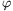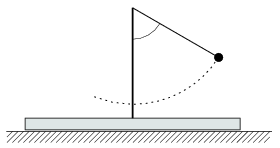Mathematical and Physical Journal
for High Schools
Issued by the MATFUND Foundation
 Already signed up? New to KöMaL?

#Problem P. 4118. (December 2008)

P. 4118. The plank of mass 2m shown in the figure can move without friction along a horizontal surface. The length of the thread of the pendulum fixed to the plank is L=20 cm, and the mass of the (point-like) bob at the end of the thread is m. The bob is displaced such that the angle between the thread and the vertical is=60o, and then it is released.

a) Find the velocity of the bob when the thread is vertical.

b) Find the velocity of the plank when the angle between the thread and the vertical is 45o.(5 pont)

Deadline expired on January 12, 2009.

### Statistics:

 122 students sent a solution. 5 points: Filep Tibor, Hartstein Máté, Lászlóffy András, Lovas Lia Izabella, Maknics András, Márkus Bence Gábor, Mayer Martin János, Somogyi József. 3 points: 44 students. 2 points: 19 students. 1 point: 9 students. 0 point: 36 students. Unfair, not evaluated: 6 solutionss.

Problems in Physics of KöMaL, December 2008